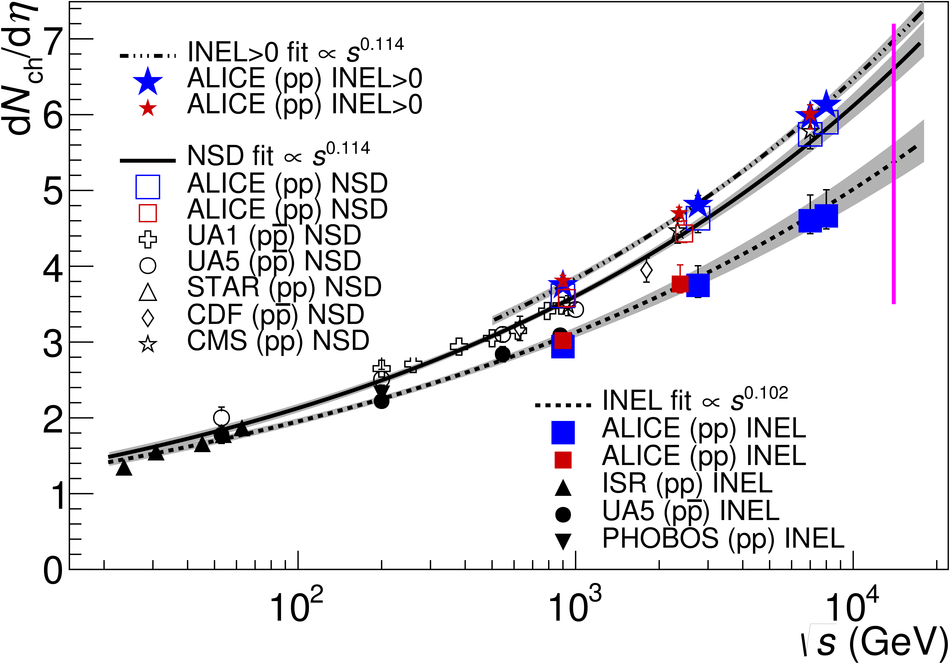# Figure 12

 Charged-particle pseudorapidity density in the pseudorapidity region $|\eta| < 0.5$ (${\rm d}\nch$/${\rm d}\eta$ at $\eta = 0$ calculated as the integral of the data over $|\eta| < 0.5$) for INEL, NSD, and INEL$>$0 collisions, as a function of the centre-of-mass energy. Lines indicate fits with a power-law dependence on $\sqrt{s}$. Grey bands represent the one standard deviation range. Data points at the same energy have been shifted horizontally for visibility. The LHC nominal centre-of-mass energy is indicated by a vertical line. Data other than from ALICE used in this figure are taken from references [25, 33, 66, 68–75].